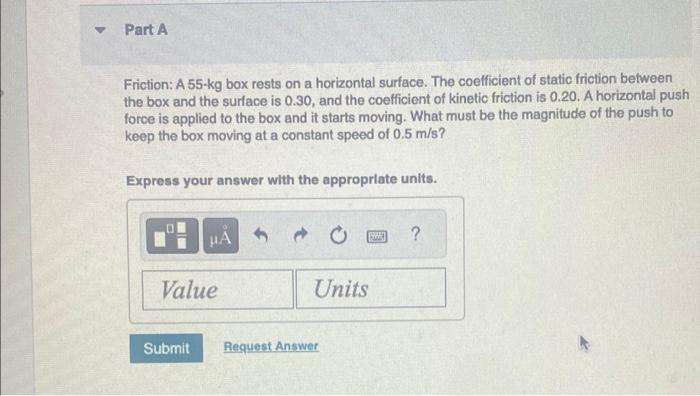# Question Friction: A $$55-\mathrm{kg}$$ box rests on a horizontal surface. The coefficient of static friction between the box and the surface is $$0.30$$, and the coefficient of kinetic friction is $$0.20$$. A horizontal push force is applied to the box and it starts moving. What must be the magnitude of the push to keep the box moving at a constant speed of $$0.5 \mathrm{~m} / \mathrm{s}$$ ? Express your answer with the appropriate units.Transcribed Image Text: Friction: A $$55-\mathrm{kg}$$ box rests on a horizontal surface. The coefficient of static friction between the box and the surface is $$0.30$$, and the coefficient of kinetic friction is $$0.20$$. A horizontal push force is applied to the box and it starts moving. What must be the magnitude of the push to keep the box moving at a constant speed of $$0.5 \mathrm{~m} / \mathrm{s}$$ ? Express your answer with the appropriate units.
Transcribed Image Text: Friction: A $$55-\mathrm{kg}$$ box rests on a horizontal surface. The coefficient of static friction between the box and the surface is $$0.30$$, and the coefficient of kinetic friction is $$0.20$$. A horizontal push force is applied to the box and it starts moving. What must be the magnitude of the push to keep the box moving at a constant speed of $$0.5 \mathrm{~m} / \mathrm{s}$$ ? Express your answer with the appropriate units.